Monday, April 27, 2009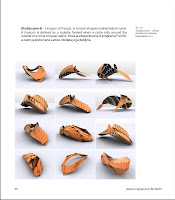x=3+cos(u+v)+cos(v)+cos(u)+sin(u); y=3+cos(u+v)+cos(v)-cos(u)+sin(u); z=cos(1.5+u)*cos(v+u)+2; 3D Surface by student Jelena Ognjanovic, course Generic Explorations 04.

Wednesday, April 8, 2009

3D Curve exploration by student Ana Jevtic, course Generic Explorations 03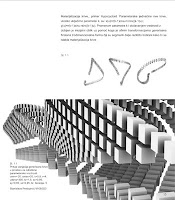3D Curve exploration by student Stanislava Predojevic, course Generic Explorations 03

Monday, April 6, 2009Mathematics in Architecture 2, student Jelena Nikolov
3^(cos(x)+cos(y)+cos(z))+4*cos(x)*cos(y)*cos(z) x,y,z range from -3 to 3
-(exp(x) + exp(y) + exp(z)) + tan(5*x)+tan(5*y)+tan(5*z) -log(12.36) x,y,z range from -5 to 5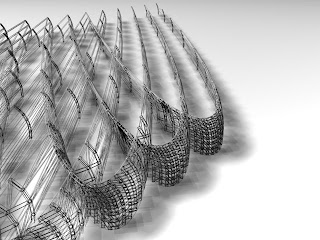3D Curve materialization by student Stevan Arsic, Course: Generic Explorations 04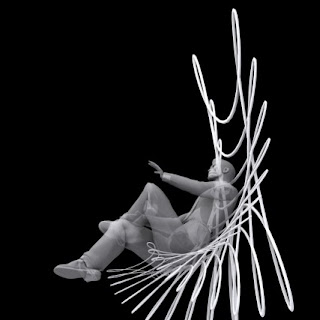3D Curve exploration by Ljiljana Mijatovic, Course: Generic Explorations 04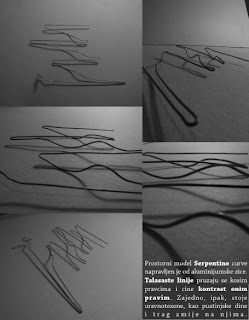3D curve - an analog model by Alexandar Kotevski

Thursday, April 2, 2009A 3D Curve generated in the Fun3D JAVA applet.
The parametric functions of the curve are:
x=30*sin(t)*t
y=30*cos(t)*t
z=10t
t ranges from 0 to 20, steps 15
The curve has been discretized (the number of steps has been slightly reduced), which gives the curve a particular quality...One of Mirjana's recent explorations of the Mandelbrot set...

3D Curve Exploration

The Fun3D software has been added to the Link collection. Congratulations to the author Bojan Mitrovic...

The Blog CreationThe generic Explorations Blog has been created... Cheers!